# Configuring a Worst Case Analysis in Multisim

Publish Date: Mar 27, 2012 | 1 Ratings | 5.00 out of 5 | Print | Submit your review

## Overview

Multisim features a comprehensive suite of SPICE analyses for examining circuit behavior. These analyses range from the basic to sophisticated. Each analysis helps you to obtain valuable information such as the effects of component tolerances and sensitivities. For each analysis you need to set settings that will inform Multisim exactly what to analyze, and how.

Multisim simplifies the procedure for an advanced analysis by providing a configuration window. This abstracts away the complexities associated with SPICE syntax and configuration of an analysis. With this window you merely need to specify the parameter values and output nodes of interest.

This tutorial is part of the National Instruments SPICE Analysis Fundamentals Series. Each tutorial in this series provides you with step-by-step instructions on how to configure and run the different SPICE analyses available in Multisim.powerful simulation and analysis while abstracting the complexity of SPICE syntax.

### 1. Introduction

Worst Case Analysis is a statistical technique that uses a “what if” approach to provide a practical result and its primary purpose is to identify the most critical components of a circuit. This analysis helps you answer the question: What will be the worst possible effects of variations in component parameters?

In Worst Case Analysis, components are varied one at a time. This allows you to calculate the sensitivity of an output variable for each component in the circuit. Finally, after each component has been varied, a final run is done with all the components parameters. This gives the worst case output.

Multisim performs Worst Case Analysis using the following process:

1. Simulation is first performed with nominal values.
2. A Sensitivity Analysis, either AC or DC, is performed to determine the sensitivity of the specified components on the output voltage or current.
3. Worst case parameters are determined by adding or subtracting the tolerance value from the nominal value.
4. Simulation is performed with the component parameter values that will produce worst case values at the output.

Assumptions: Applied to an analog circuit, DC and small-signal. Models are linearized.

### 2. Running Worst Case Analysis

Consider the Wien-bridge oscillator circuit shown in Figure 1. The feedback to the non-inverting pin is designed to stabilize the amplitude of the oscillator, thus R1-R4 must be chosen carefully to ensure this circuit will oscillate. The following two conditions must be met: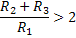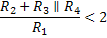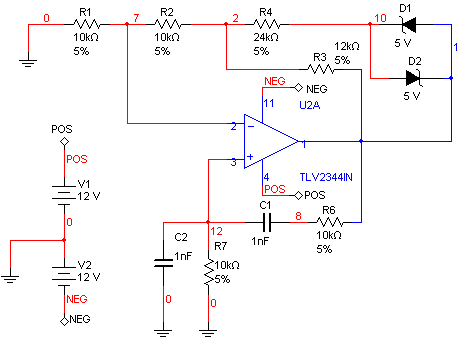Figure 1. Wien-bridge oscillator circuit.

In this example you will use Worst Case Analysis to determine if the circuit will continue to oscillate under the worst conditions.

Complete the following steps to configure and run a Worst Case Analysis:

2. Open the Oscilloscope front panel and run the simulation. Observe the sinewave generated by the oscillator circuit.
3. Stop the simulation.
4. Select Simulate»Analyses»Worst Case. In the Model tolerance list tab you can select which tolerance parameters will be used. Note that a tolerance for R1 has already been added.
5. Click Add tolerance. The Tolerance dialog window appears. Configure this window as shown in the following figure: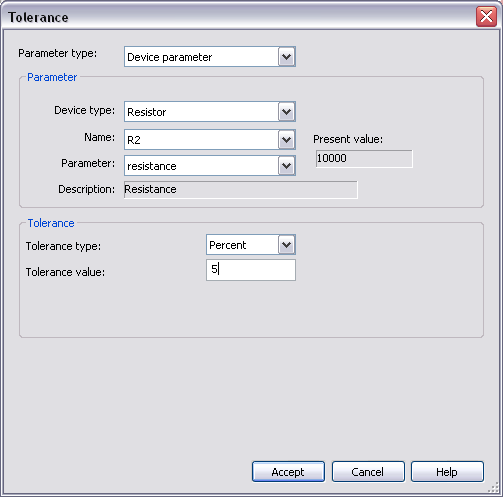1. Click Accept. R2 is added to the list of tolerances.
2. Repeat steps 5 and 6 to add tolerances for R3 and R4. The dialog window will look like the following figure: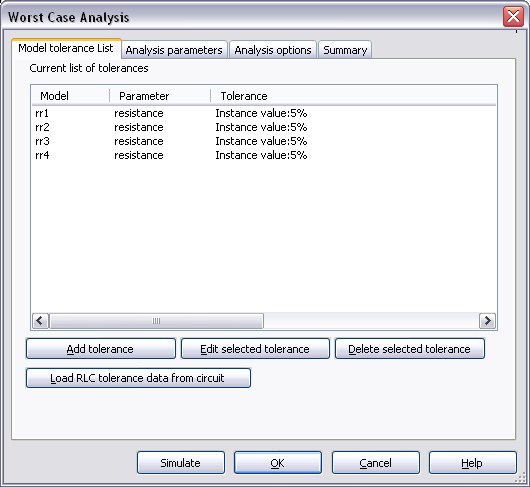Figure 3. Model tolerance list.

1. Select the Analysis Parameters tab.
2. Set the analysis settings as shown in Figure 4. Refer to Table 1 for details on the different analysis parameters.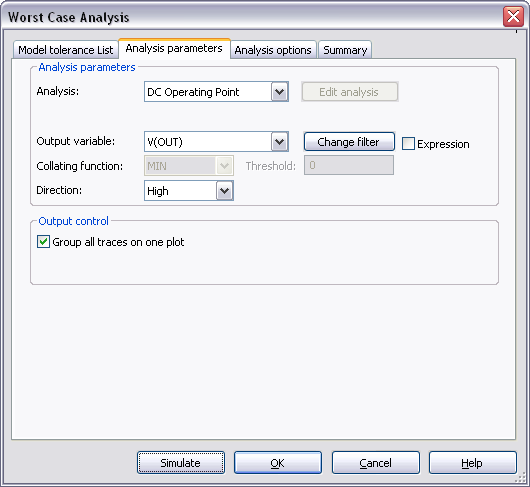Figure 4. Worst Case Analysis parameters.

Table 1. Parameters used in Worst Case Analysis.

 Parameter Meaning Analysis Defines the type of analysis required. This can be DC Operating Point or AC Analysis. If you select an AC Analysis, the Edit Analysis button will enabled. Click on this button to configure Frequency Parameters. Output variable Output variable to be monitored. Collating Function Specifies the operation to be performed on the values of the output variable to reduce these to a single number that will be used in the sensitivity calculations (this option is enabled when you select an AC Analysis). There are five functions available: MAX: Finds the maximum value of each waveform. MIN: Finds the minimum value of each waveform. RISE_EDGE (value):  Finds the first occurrence of the waveform crossing above the threshold (value). FALL_EDGE (value): Finds the first occurrence of the waveform crossing below the threshold (value). FREQUENCY: Finds the value at a specified frequency. Direction Describes what kind of output will be generated and limits the range over which the Collating Function operates. Multisim supports the option: HI/LOW: Specifies which direction the worst case run is to go relative to the nominal run. Change Filter Filters the variables displayed to include internal nodes (such as nodes inside a BJT model or inside a SPICE subcircuit), open pins, as well as output variables from any sub-modules contained in the circuit. If you click on the Expression checkbox, the Change Filter button will change to Edit Expression; select this option if you need to create a custom expression.

Note: In SPICE, the command that performs a Worst Case Analysis has the following form:

.WCASE <ANALYSIS> <OUTPUT_VARIABLE> <FUNCTION> [OPTION]

Note that these are the same parameters that were defined in Table 1, however, in Multisim you do not have to worry about the SPICE syntax.

1. Click Simulate. Results are displayed in Figure 5.

The Worst Case Analysis section shows the DC Operating Point at V(OUT) for both nominal and worst case. The Run Log Descriptions section displays the resistance values required to achieve the greatest difference from the nominal value.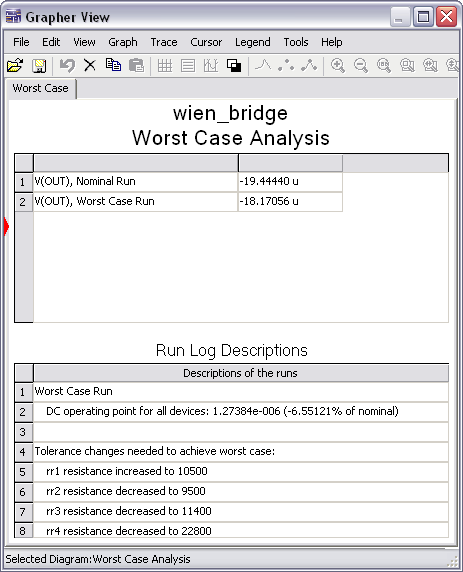Figure 5. Worst Case Analysis results.

Inserting  the output results in the two conditions for oscillation: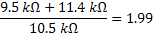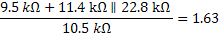As you can see, only one condition is met, therefore, the circuit may fail if the components tolerances are not carefully chosen. Try entering the worst case values in the Wien-bridge circuit. Does it oscillate?

For AC circuits, the Worst Case Analysis generates separate plots for the nominal and worst case runs. A list of the components and their worst case values appears in tabular form. You can find an additional example file (speech_filter.ms11) in the Downloads section. In this case, resistors and capacitors of the Speech Filter circuit are varied to see the effects in the AC response.

Entering Expressions in Analyses in Multisim

SPICE Analysis Fundamentals

Circuit Design Technical Library

Join the NI Circuit Design Community